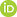Full Text:<1971>

Summary:<1516>

CLC number: TP13

On-line Access: 2017-12-04

Revision Accepted: 2016-10-15

Crosschecked: 2017-11-01

Cited: 0

Clicked: 5741

Citations:  Bibtex RefMan EndNote GB/T7714ORCID:

Xue-song Chen

http://orcid.org/0000-0001-9530-0644

### -   Go to

Article info.
 Frontiers of Information Technology & Electronic Engineering  2017 Vol.18 No.10 P.1479-1487 http://doi.org/10.1631/FITEE.1601101Galerkin approximation with Legendre polynomials for a continuous-time nonlinear optimal control problem

 Author(s):  Xue-song Chen Affiliation(s):  School of Applied Mathematics, Guangdong University of Technology, Guangzhou 510006, China Corresponding email(s):   chenxs@gdut.edu.cn Key Words:  Generalized Hamilton-Jacobi-Bellman equation, Nonlinear optimal control, Galerkin approximation, Legendre polynomials Share this article to： More <<< Previous Article|Next Article >>>

Xue-song Chen. Galerkin approximation with Legendre polynomials for a continuous-time nonlinear optimal control problem[J]. Frontiers of Information Technology & Electronic Engineering, 2017, 18(10): 1479-1487.

@article{title="Galerkin approximation with Legendre polynomials for a continuous-time nonlinear optimal control problem",
author="Xue-song Chen",
journal="Frontiers of Information Technology & Electronic Engineering",
volume="18",
number="10",
pages="1479-1487",
year="2017",
publisher="Zhejiang University Press & Springer",
doi="10.1631/FITEE.1601101"
}

%0 Journal Article
%T Galerkin approximation with Legendre polynomials for a continuous-time nonlinear optimal control problem
%A Xue-song Chen
%J Frontiers of Information Technology & Electronic Engineering
%V 18
%N 10
%P 1479-1487
%@ 2095-9184
%D 2017
%I Zhejiang University Press & Springer
%DOI 10.1631/FITEE.1601101

TY - JOUR
T1 - Galerkin approximation with Legendre polynomials for a continuous-time nonlinear optimal control problem
A1 - Xue-song Chen
J0 - Frontiers of Information Technology & Electronic Engineering
VL - 18
IS - 10
SP - 1479
EP - 1487
%@ 2095-9184
Y1 - 2017
PB - Zhejiang University Press & Springer
ER -
DOI - 10.1631/FITEE.1601101

Abstract:
We investigate the use of an approximation method for obtaining near-optimal solutions to a kind of nonlinear continuous-time (CT) system. The approach derived from the galerkin approximation is used to solve the generalized Hamilton-Jacobi-Bellman (GHJB) equations. The galerkin approximation with legendre polynomials (GALP) for GHJB equations has not been applied to nonlinear CT systems. The proposed GALP method solves the GHJB equations in CT systems on some well-defined region of attraction. The integrals that need to be computed are much fewer due to the orthogonal properties of legendre polynomials, which is a significant advantage of this approach. The stabilization and convergence properties with regard to the iterative variable have been proved. Numerical examples show that the update control laws converge to the optimal control for nonlinear CT systems.

### Reference

Aguilar, C.O., Krener, A.J., 2014. Numerical solutions to the Bellman equation of optimal control. J. Optim. Theory Appl., 160(2):527-552.Bardi, M., Capuzzo-Dolcetta, I., 1997. Optimal Control and Viscosity Solutions of Hamilton-Jacobi-Bellman Equations. Birkhäuser Boston, Inc., Boston, MA (with appendices by Maurizio Falcone and Pierpaolo Soravia).Beard, R.W., Saridis, G.N., Wen, J.T., 1996. Improving the performance of stabilizing controls for nonlinear systems. IEEE Contr. Syst. Mag., 16(5):27-35.Beard, R.W., Saridis, G.N., Wen, J.T., 1997. Galerkin approximations of the generalized Hamilton-Jacobi-Bellman equation. Automatica, 33(12):2159-2177.Bellman, R., 1957. Dynamic Programming. Princeton University Press, New Jersey, USA.

Cacace, S., Cristiani, E., Falcone, M., et al., 2012. A patchy dynamic programming scheme for a class of Hamilton-Jacobi-Bellman equations. SIAM J. Sci. Comput., 34(5):A2625-A2649.Canuto, C., Hussaini, M.Y., Quarteroni, A., et al., 1988. Spectral Methods in Fluid Dynamics. Springer-Verlag, New York, USA.

Gong, Q., Kang, W., Ross, I.M., 2006. A pseudospectral method for the optimal control of constrained feedback linearizable systems. IEEE Trans. Autom. Contr., 51(7):1115-1129.Govindarajan, N., de Visser, C.C., Krishnakumar, K., 2014. A sparse collocation method for solving time-dependent HJB equations using multivariate B-splines. Automatica, 50(9):2234-2244.Isidori, A., 2013. Nonlinear Control Systems. Springer Science & Business Media.

Kirk, D.E., 2012. Optimal Control Theory: an Introduction. Courier Corporation.

Kleinman, D., 1968. On an iterative technique for Riccati equation computations. IEEE Trans. Autom. Contr., 13(1):114-115.Lews, F., Syrmos, V., 1995. Optimal Control. Wiley, New Jersey, USA.

Luo, B., Wu, H.N., Huang, T., et al., 2014. Data-based approximate policy iteration for affine nonlinear continuous-time optimal control design. Automatica, 50(12):3281-3290.Luo, B., Huang, T., Wu, H.N., et al., 2015a. Data-driven Hinfty control for nonlinear distributed parameter systems. IEEE Trans. Neur. Netw. Learn. Syst., 26(11):2949-2961.Luo, B., Wu, H.N., Huang, T., 2015b. Off-policy reinforcement learning for Hinfty control design. IEEE Trans. Cybern., 45(1):65-76.Luo, B., Wu, H.N., Li, H.X., 2015c. Adaptive optimal control of highly dissipative nonlinear spatially distributed processes with neuro-dynamic programming. IEEE Trans. Neur. Netw. Learn. Syst., 26(4):684-696.Markman, J., Katz, I.N., 2000. An iterative algorithm for solving Hamilton-Jacobi type equations. SIAM J. Sci. Comput., 22(1):312-329.Sakamoto, N., van der Schaft, A.J., 2008. Analytical approximation methods for the stabilizing solution of the Hamilton-Jacobi equation. IEEE Trans. Autom. Contr., 53(10):2335-2350.Saridis, G.N., Lee, C.S.G., 1979. An approximation theory of optimal control for trainable manipulators. IEEE Trans. Syst. Man Cybern., 9(3):152-159.Smears, I., Süli, E., 2014. Discontinuous Galerkin finite element approximation of Hamilton-Jacobi-Bellman equations with Cordes coefficients. SIAM J. Numer. Anal., 52(2):993-1016.Wu, H.N., Luo, B., 2012. Neural network based online simultaneous policy update algorithm for solving the HJI equation in nonlinear control. IEEE Trans. Neur. Netw. Learn. Syst., 23(12):1884-1895.Yu, J., Jiang, Z.P., 2015. Global adaptive dynamic programming for continuous-time nonlinear systems. IEEE Trans. Autom. Contr., 60(11):2917-2929.